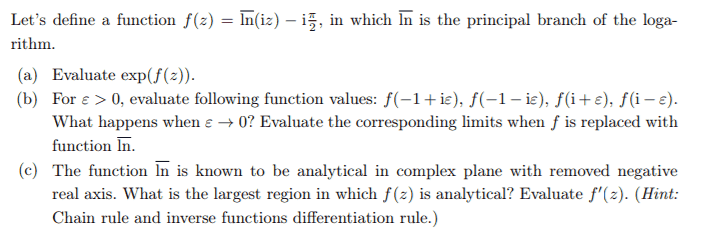# Finding analyticity of a complex function involving ln(iz)

• tixi

#### tixi

Homework Statement
The function ln (principal value of ln) is known to be analytical in complex plane with removed negative real axis. What is the largest region in which f(z)=ln(iz)-i pi/2 is analytical? Evaluate f′(z).
Relevant Equations
Principal value of the logarithm: ln(z) = ln(r) + iArg(z)
Chain rule for complex functions
Inverse functions differentiation rule
Hey everyone! I got stuck with one of my homework questions. I don't 100% understand the question, let alone how I should get started with the problem.

The picture shows the whole problem, but I think I managed doing the a and b parts, just got stuck with c. How do I find the largest region in which f(z) is analytical and how do I get started trying to differentiate it? Do the differentiation rules for the real ln translate to the complex one?Thank you so much! I never got a notification that my thread was answered, but this still helped! The exercise was explained eventually but it was very abstract and they just gave a very simple answer, so the motivation you provided was still helpful! Thanks•